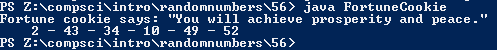# Assignment #55

## Code

```    /// Name: Kabir Virk
/// Period: 6
/// Date Finished: 12/2/2015

import java.util.Random;

{
public static void main(String[] args)
{
Random r = new Random();

int fortune = 1 + r.nextInt(6);
int fort1 = 1 + r.nextInt(54);
int fort2 = 1 + r.nextInt(54);
int fort3 = 1 + r.nextInt(54);
int fort4 = 1 + r.nextInt(54);
int fort5 = 1 + r.nextInt(54);
int fort6 = 1 + r.nextInt(54);

if (fortune == 1)
else if (fortune == 2)
else if (fortune == 3)
System.out.println("Fortune cookie says: \"Success is around the corner.\"");
else if (fortune == 4)
System.out.println("Fortune cookie says: \"A family member nearby is waitinf for yo.\"");
else if (fortune == 5)